# 1 Goal of this vignette

Proteomics data suffer from a high rate of missing values, which need to be accounted for. Different methods have been applied to deal with this issue, including multiple imputation methods (see for example Lazar et al). The different options to deal with missing values in DEP are described in this vignette.

# 2 Simulate data

To exemplify the missing value handling, we work with a simulated dataset. This is very useful, because we know the ground truth of our dataset, meaning we know which proteins are belonging to the background (null distribution) and which proteins are differentially expressed between the control and sample conditions.

## 2.1 Generate intensity values

We generate a dataset with 3300 proteins, of which 300 proteins are differentially expressed. The variables used to generate the data are depicted below.

``````# Variables
replicates = 3
bg_proteins = 3000
DE_proteins = 300
log2_mean_bg = 27
log2_sd_bg = 2
log2_mean_DE_control = 25
log2_mean_DE_treatment = 30
log2_sd_DE = 2``````
``````# Loading DEP and packages required for data handling
library("DEP")
library("dplyr")
library("tidyr")
library("purrr")
library("ggplot2")
library("SummarizedExperiment")

# Background data (sampled from null distribution)
sim_null <- data_frame(
name = paste0("bg_", rep(1:bg_proteins, rep((2*replicates), bg_proteins))),
ID = rep(1:bg_proteins, rep((2*replicates), bg_proteins)),
var = rep(c("control_1", "control_2", "control_3",
"treatment_1", "treatment_2", "treatment_3"), bg_proteins),
val = 2^rnorm(2*replicates*bg_proteins, mean = log2_mean_bg, sd = log2_sd_bg))``````
``````## Warning: `data_frame()` is deprecated, use `tibble()`.
## This warning is displayed once per session.``````
``````# Data for DE proteins (sampled from alternative distribution)
sim_diff <- rbind(
data_frame(
name = paste0("DE_", rep(1:DE_proteins, rep(replicates, DE_proteins))),
ID = rep((bg_proteins+1):(bg_proteins+DE_proteins),
rep(replicates, DE_proteins)),
var = rep(c("control_1", "control_2", "control_3"), DE_proteins),
val = 2^rnorm(replicates*DE_proteins,
mean = log2_mean_DE_control, sd = log2_sd_DE)),
data_frame(
name = paste0("DE_", rep(1:DE_proteins, rep(replicates, DE_proteins))),
ID = rep((bg_proteins+1):(bg_proteins+DE_proteins),
rep(replicates, DE_proteins)),
var = rep(c("treatment_1", "treatment_2", "treatment_3"), DE_proteins),
val = 2^rnorm(replicates*DE_proteins,
mean = log2_mean_DE_treatment, sd = log2_sd_DE)))

# Combine null and DE data
sim <- rbind(sim_null, sim_diff) %>%
arrange(ID)

# Generate experimental design
experimental_design <- data_frame(
label = colnames(sim)[!colnames(sim) %in% c("name", "ID")],
condition = c(rep("control", replicates),
rep("treatment", replicates)),
replicate = rep(1:replicates, 2))``````

## 2.2 Introduce missing values

Data can be missing at random (MAR) or missing not at random (MNAR). MAR means that values are randomly missing from all samples. In the case of MNAR, values are missing in specific samples and/or for specific proteins. For example, certain proteins might not be quantified in specific conditions, because they are below the detection limit in these specific samples.

To mimick these two types of missing values, we introduce missing values randomly over all data points (MAR) and we introduce missing values in the control samples of 100 differentially expressed proteins (MNAR). The variables used to introduce missing values are depicted below.

``````# Variables
MAR_fraction = 0.05
MNAR_proteins = 100``````
``````# Generate a MAR matrix
MAR_matrix <- matrix(
data = sample(c(TRUE, FALSE),
size = 2*replicates*(bg_proteins+DE_proteins),
replace = TRUE,
prob = c(MAR_fraction, 1-MAR_fraction)),
nrow = bg_proteins+DE_proteins,
ncol = 2*replicates)

# Introduce missing values at random (MAR)
controls <- grep("control", colnames(sim))
treatments <- grep("treatment", colnames(sim))
sim[, c(controls, treatments)][MAR_matrix] <- 0
sim\$MAR <- apply(MAR_matrix, 1, any)

# Introduce missing values not at random (MNAR)
DE_protein_IDs <- grep("DE", sim\$name)
sim[DE_protein_IDs[1:MNAR_proteins], controls] <- 0
sim\$MNAR <- FALSE
sim\$MNAR[DE_protein_IDs[1:MNAR_proteins]] <- TRUE``````

## 2.3 Generate a SummarizedExperiment

The data is stored in a SummarizedExperiment, as described in the ‘Introduction to DEP’ vignette.

``````# Generate a SummarizedExperiment object
sim_unique_names <- make_unique(sim, "name", "ID", delim = ";")
se <- make_se(sim_unique_names, c(controls, treatments), experimental_design)``````

# 3 Filter proteins based on missing values

A first consideration with missing values is whether or not to filter out proteins with too many missing values.

## 3.1 Visualize the extend of missing values

The number of proteins quantified over the samples can be visualized to investigate the extend of missing values.

``````# Plot a barplot of the protein quantification overlap between samples
plot_frequency(se)``````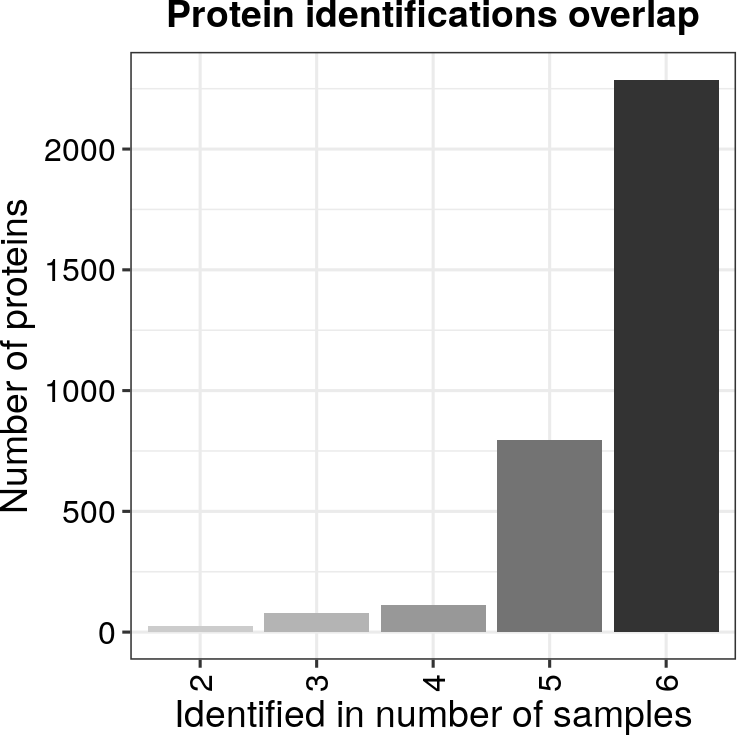Many proteins are quantified in all six samples and only a small subset of proteins were detected in less than half of the samples.

## 3.2 Filter options

We can choose to not filter out any proteins at all, filter for only the proteins without missing values, filter for proteins with a certain fraction of quantified samples, and for proteins that are quantified in all replicates of at least one condition.

``````# No filtering
no_filter <- se

# Filter for proteins that are quantified in all replicates of at least one condition
condition_filter <- filter_proteins(se, "condition", thr = 0)

# Filter for proteins that have no missing values
complete_cases <- filter_proteins(se, "complete")

# Filter for proteins that are quantified in at least 2/3 of the samples.
frac_filtered <- filter_proteins(se, "fraction", min = 0.66)``````

To check the consequences of filtering, we calculate the number of background and DE proteins left in the dataset.

``````# Function to extract number of proteins
number_prots <- function(se) {
names <- rownames(get(se))
data_frame(Dataset = se,
bg_proteins = sum(grepl("bg", names)),
DE_proteins = sum(grepl("DE", names)))
}

# Number of bg and DE proteins still included
objects <- c("no_filter",
"condition_filter",
"complete_cases",
"frac_filtered")

map_df(objects, number_prots)``````
``````## # A tibble: 4 x 3
##   Dataset          bg_proteins DE_proteins
##   <chr>                  <int>       <int>
## 1 no_filter               3000         300
## 2 condition_filter        2937         267
## 3 complete_cases          2147         141
## 4 frac_filtered           2997         200``````

For our simulated dataset, filtering for complete cases drastically reduces the number of proteins, only keeping half of the DE proteins. Filtering protein to be quantified in at least 2/3 of the samples, keeps many more DE proteins and filters out all proteins with values MNAR. Filtering for proteins quantified in all replicates of at least one condition only filters out a minimal amount of DE proteins.

## 3.3 Scaling and variance stabilization

The data is scaled and variance stabilized using vsn.

``````# Scale and variance stabilize
no_filter <- normalize_vsn(se)
condition_filter <- normalize_vsn(condition_filter)
complete_cases <- normalize_vsn(complete_cases)
frac_filtered <- normalize_vsn(frac_filtered)``````
``````# Mean versus Sd plot
meanSdPlot(no_filter)``````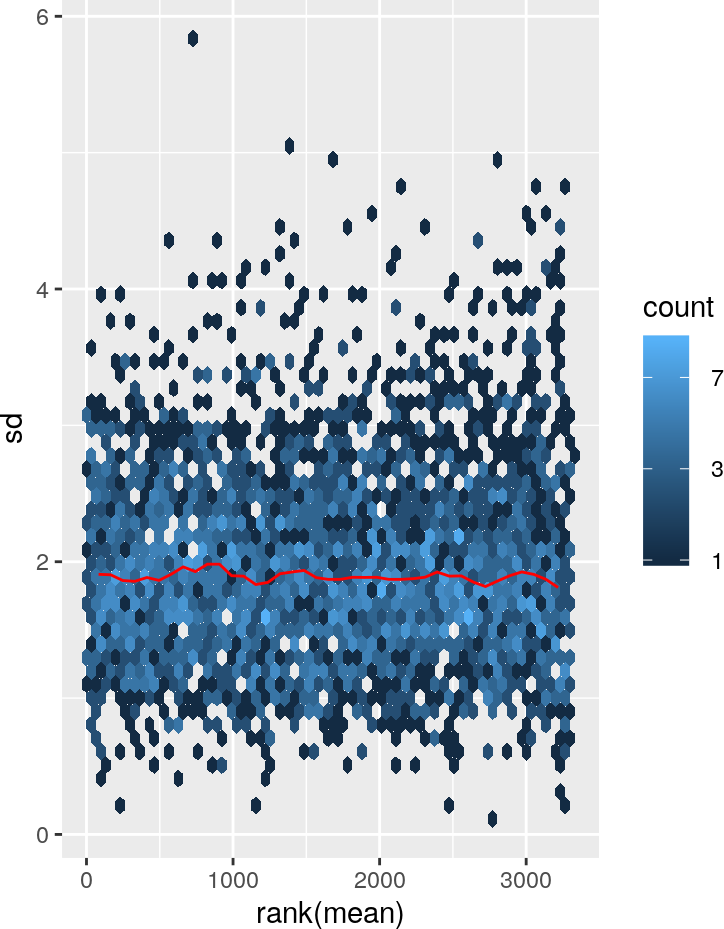# 4 Data imputation of missing data

A second important consideration with missing values is whether or not to impute the missing values.

MAR and MNAR (see Introduce missing values) require different imputation methods. See the MSnbase vignette and more specifically the impute function descriptions for detailed information.

## 4.1 Explore the pattern of missing values

To explore the pattern of missing values in the data, a heatmap can be plotted indicating whether values are missing (0) or not (1). Only proteins with at least one missing value are visualized.

``````# Plot a heatmap of proteins with missing values
plot_missval(no_filter)``````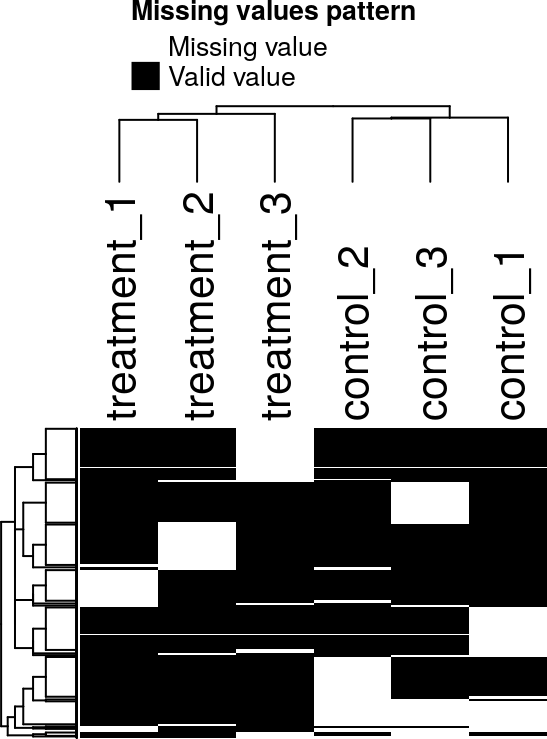The missing values seem to be randomly distributed across the samples (MAR). However, we do note a block of values that are missing in all control samples (bottom left side of the heatmap). These proteins might have missing values not at random (MNAR).

To check whether missing values are biased to lower intense proteins, the densities and cumulative fractions are plotted for proteins with and without missing values.

``````# Plot intensity distributions and cumulative fraction of proteins
# with and without missing values
plot_detect(no_filter)``````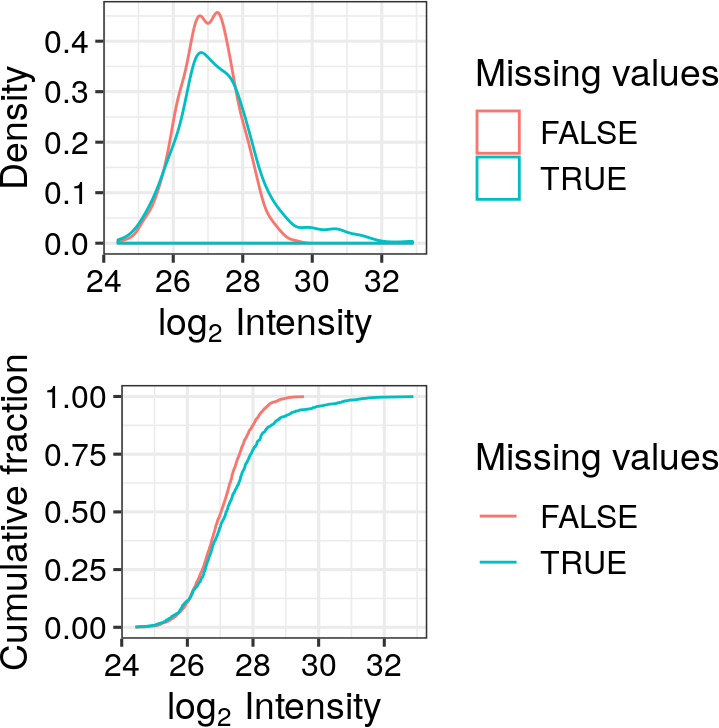In our example data, there is no clear difference between the two distributions.

## 4.2 Imputation options

DEP borrows the imputation functions from MSnbase. See the MSnbase vignette and more specifically the impute function description for more information on the imputation methods.

``````# All possible imputation methods are printed in an error, if an invalid function name is given.
impute(no_filter, fun = "")``````
``## Error in match.arg(fun): 'arg' should be one of "bpca", "knn", "QRILC", "MLE", "MinDet", "MinProb", "man", "min", "zero", "mixed", "nbavg"``

Some examples of imputation methods.

``````# No imputation
no_imputation <- no_filter

# Impute missing data using random draws from a
# Gaussian distribution centered around a minimal value (for MNAR)
MinProb_imputation <- impute(no_filter, fun = "MinProb", q = 0.01)

# Impute missing data using random draws from a
# manually defined left-shifted Gaussian distribution (for MNAR)
manual_imputation <- impute(no_filter, fun = "man", shift = 1.8, scale = 0.3)

# Impute missing data using the k-nearest neighbour approach (for MAR)
knn_imputation <- impute(no_filter, fun = "knn", rowmax = 0.9)``````

The effect of data imputation on the distributions can be visualized.

``````# Plot intensity distributions before and after imputation
plot_imputation(no_filter, MinProb_imputation,
manual_imputation, knn_imputation)``````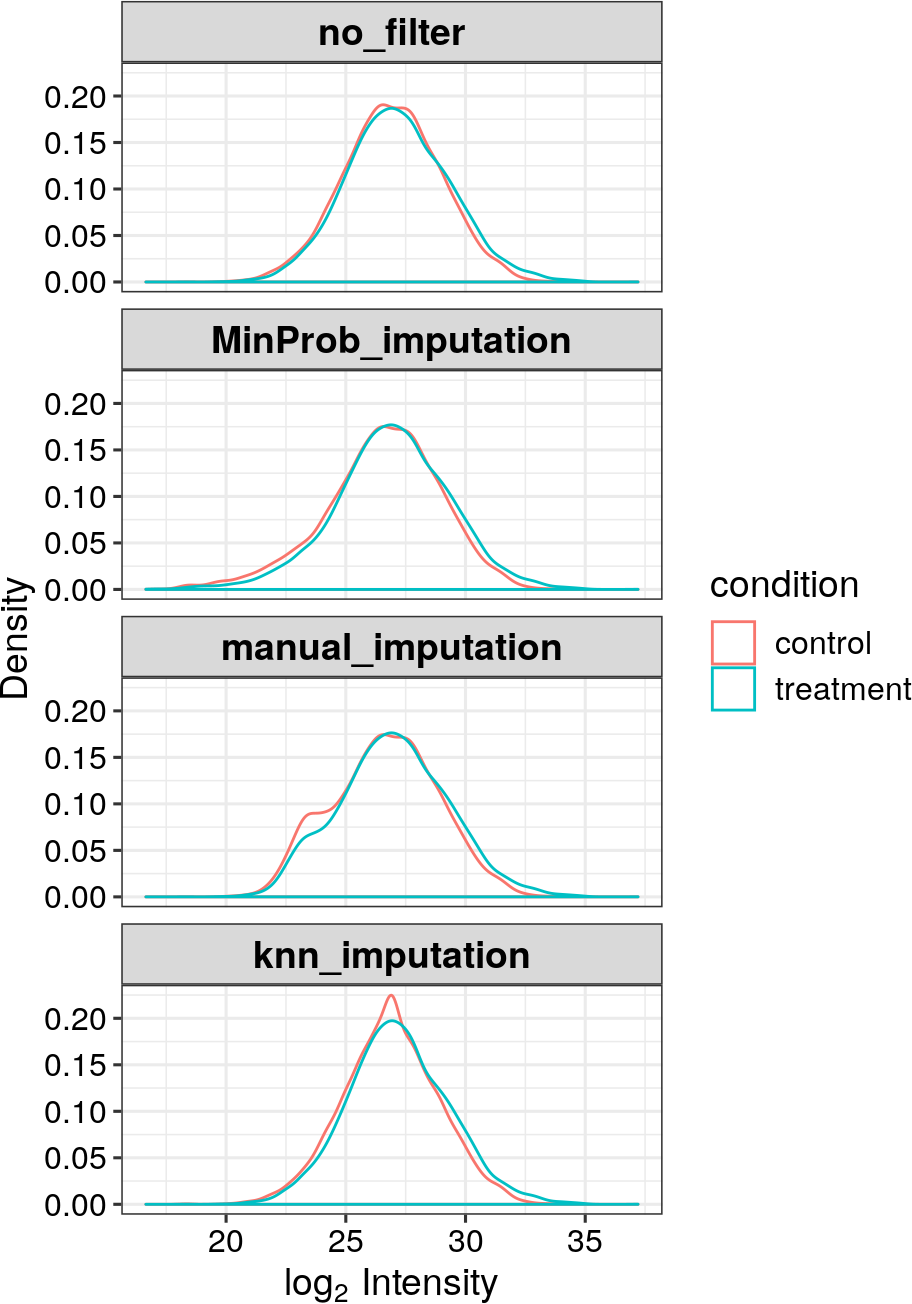### 4.3.1 Mixed imputation on proteins (rows)

One can also perform a mixed imputation on the proteins, which uses a MAR and MNAR imputation method on different subsets of proteins. First, we have to define a logical vector defining the rows that are to be imputed with the MAR method. Here, we consider a protein to have missing values not at random (MNAR) if it has missing values in all replicates of at least one condition.

``````# Extract protein names with missing values
# in all replicates of at least one condition
proteins_MNAR <- get_df_long(no_filter) %>%
group_by(name, condition) %>%
summarize(NAs = all(is.na(intensity))) %>%
filter(NAs) %>%
pull(name) %>%
unique()

# Get a logical vector
MNAR <- names(no_filter) %in% proteins_MNAR

# Perform a mixed imputation
mixed_imputation <- impute(
no_filter,
fun = "mixed",
randna = !MNAR, # we have to define MAR which is the opposite of MNAR
mar = "knn", # imputation function for MAR
mnar = "zero") # imputation function for MNAR``````

### 4.3.2 Mixed imputation on samples (columns)

Additionally, the imputation can also be performed on a subset of samples. To peform a sample specific imputation, we first need to transform our SummarizedExperiment into a MSnSet object. Subsequently, we imputed the controls using the “MinProb” method and the samples using the “knn” method.

``````# SummarizedExperiment to MSnSet object conversion
sample_specific_imputation <- no_filter
MSnSet <- as(sample_specific_imputation, "MSnSet")

# Impute differently for two sets of samples
MSnSet_imputed1 <- MSnbase::impute(MSnSet[, 1:3], method = "MinProb")
MSnSet_imputed2 <- MSnbase::impute(MSnSet[, 4:6], method = "knn")

# Combine into the SummarizedExperiment object
assay(sample_specific_imputation) <- cbind(
MSnbase::exprs(MSnSet_imputed1),
MSnbase::exprs(MSnSet_imputed2))``````

The effect of data imputation on the distributions can be visualized.

``````# Plot intensity distributions before and after imputation
plot_imputation(no_filter, mixed_imputation, sample_specific_imputation)``````